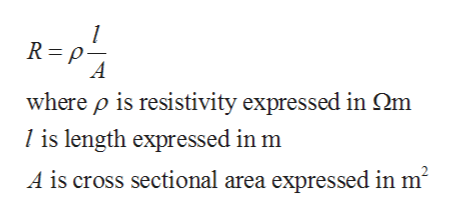airframe electrical systemQuestion: What four factors affect the resistance of wires?(Source: FAA AC 43.13-1B/2B)

Question

airframe electrical system

Question: What four factors affect the resistance of wires?

(Source: FAA AC 43.13-1B/2B)

Step 1

Resistance is the basic nature of any substance. It is the nature of material to oppose the flow of current.

SI unit of resistance is ohm.

Practically all the wires possess some resistance which can be calculated by the basic formulahelp_outlineImage Transcriptionclose1 R p A where p is resistivity expressed in Qm Iis length expressed in m A is cross sectional area expressed in m2 fullscreen
Step 2

The resistance of the wire mainly depends on the following four factors:

1. Length
2. Are...

Want to see the full answer?

See Solution

Want to see this answer and more?

Our solutions are written by experts, many with advanced degrees, and available 24/7

See Solution
Tagged in

Electrical Engineering